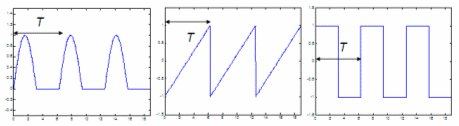Fourier Analysis - introduction from a practical point of view

In calculus or mathematics, Fourier analysis is a topic which grew from the study of Fourier series. This field began with the study of the way periodic or general functions might be represented by summations of simpler trigonometric functions (sine or cosine series).

Fourier analysis has many applications in science, physics, digital signal processing, imaging, numerical analysis, acoustics... and in many other areas.

We are going to comment on some electronic applications, where there are two emphasized domains: analysis in time domain, to find the voltages or currents at specific moments, and the analysis in the frequency domain, to study (in order to possibly manipulate) the frequencies present in a given signal.

Fourier series

This figure shows a sine wave with a peak value Vp and a period T.When AC analysis is presented in circuit theory courses, they usually focus on the sine wave because it helps understand many effects. Based on those effects or behaviors of electronic components we can start the study of non-sinusoidal signals.

Periodic waves

The shapes below (a half-sine, a sawtooth and a square wave) complete their basic form over a period T, and each cycle is an exact copy of the first period or cycle. This kind of waveform with repetitive cycles is called a periodic wave with period T.The addition of sine waves with appropriate amplitudes and phases can represent triangular waves, or a different combination of sine series and waves can produce a sawtooth signal, and another different combination may produce a half-wave signal. In other words, any periodic wave is a superposition of sine waves.

The sine waves are harmonically related, which means that the frequencies are harmonics (or multiples) of a fundamental (lowest) frequency. Given a periodic wave, you can find the period T when measured with an oscilloscope. The reciprocal of T is equal to the fundamental frequency.

In formulas:

The fundamental frequency f1 = 1 / T
The second harmonic has a frequency f2 = 2f1
The third harmonic has a frequency f3 = 3f1

In general, the nth harmonic has a frequency
fn = nf1

Formulas for the Fourier seriesExpressed in words, the figure above means:

periodic wave = DC component +
first harmonic +
second harmonic +
... +
nth harmonic

In mathematical terms:

v = V0 + V1 sin(wt + Ф1) + V2 sin(2wt + Ф2) + ... + Vn sin(nwt + Фn)

This famous equation is called the Fourier series, and it means that a periodic wave is a superposition of harmonically related sine waves.

Voltage v is the value of the periodic wave at any instant, this value can be calculated by adding the DC component and the instant values of all the the harmonics. The first term in the Fourier series is V0, which is a constant and represents the DC component. The coefficients V1, V2... Vn are the peak values of the harmonics. The angular frequency w is equal to 2πf, so you can see that each term in the Fourier series represents the next higher harmonic.

Harmonic waves theoretically continue to infinity. However, five to ten terms are often enough to represent a periodic signal within an accuracy of 5%. With the right combination of magnitudes, angles and phases, it is possible to reproduce any periodic waveform.

Now, let’s do a brief experiment with our numerical software. You can see how to produce a square wave, here.

We can implement this code:

% Define and plot one cycle of a square wave
x = 0 : .01 : 2*pi;
y = square(x);
plot(x,y)
axis([min(x) max(x) min(y)-.5 max(y)+.5])

% Plot f1 and f3 and add them up
figure
y1 = sin(x);
y2 = .2*sin(3*x);
plot(x,y1,
'b', x,y2,'b:', x, y1+y2, 'g', 'linewidth', 2)
axis([min(x) max(x) min(y)-.5 max(y)+.5])

We get two figures:The figure on the left is a plain square wave. We want to find an equivalent of that periodic wave by utilizing only sines. The figure on the right represents our first attempt to do it. We use the first and third harmonics of the original wave, we add them up and show the results. We can clearly see that the green line is getting closer to a square wave. In order to produce a better approximation, we’d need to adjust our amplitudes and include more terms in the series.

In the next article, we’re going to explore three very common periodic waves and the specific Fourier Series to produce them.

Reference:

Malvino, A. P.; Electronic Principles; 2nd. edition; McGraw-Hill Co., 1979.

From 'Fourier Analysis ' to home

From 'Fourier Analysis ' to Calculus Problems

 Top Periodic Waves Taylor Expansions Harmonic Series Fourier Series